# Free Printable Math Worksheets

1. Worksheets >
2. Math

Convert your class from a bunch of passive learners to active learners beaming with energy as they use our worksheets and transit between watching and doing. Worksheets have a widespread use in math curriculum, and therefore, teachers love to incorporate them, but a lot of time and effort go into creating them. Do away with this barrier; and save your time and effort with our no-prep print-ready worksheets. Introduce key math topics and provide adequate practice with this compilation of free printable math worksheets for students of kindergarten through grade 12.

### Number Sense and Operations Worksheets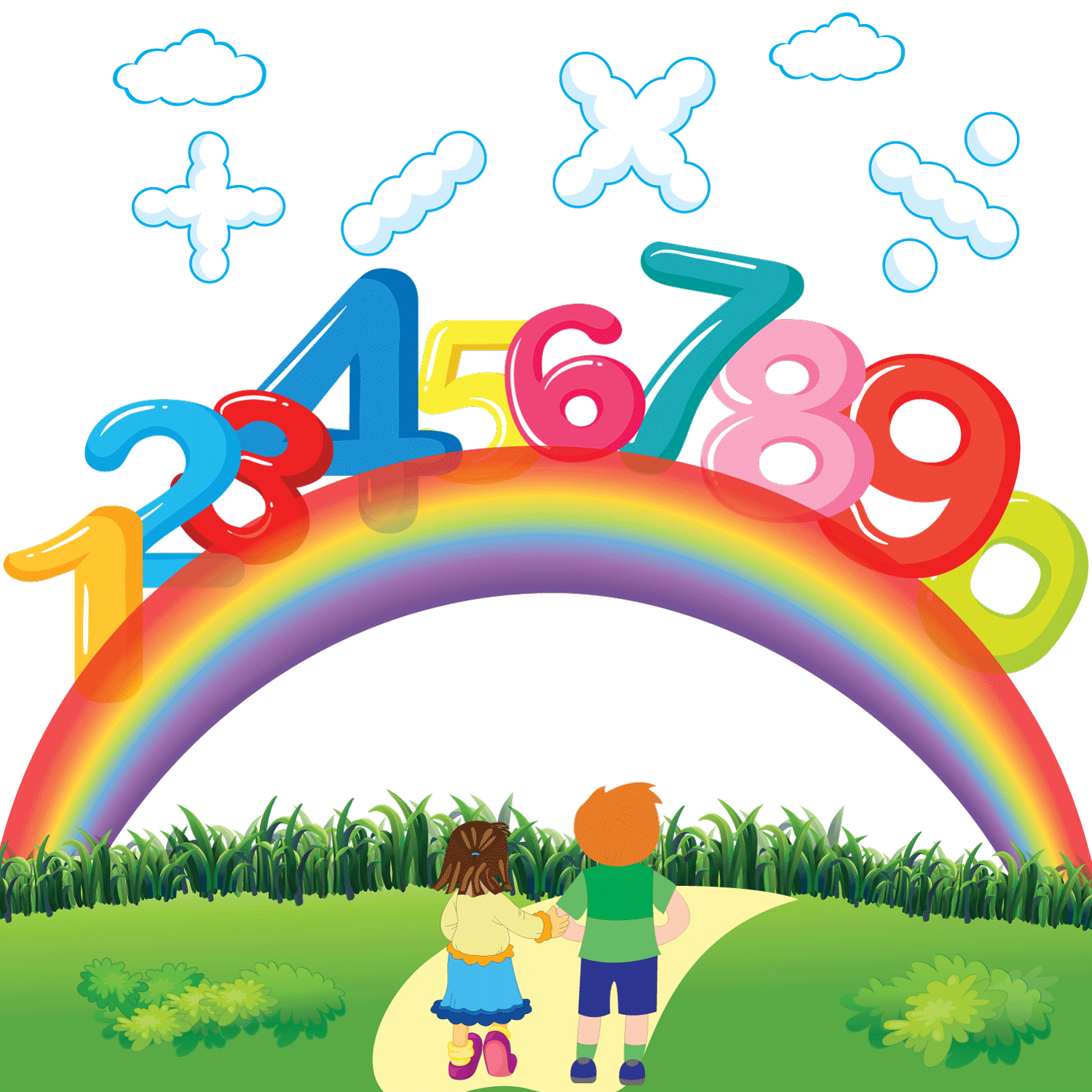The best way to instill a sense of numbers in kids is to let them practice and make connections between numbers and their quantities. Carrying out arithmetic operations is yet another skill that kids develop with this interesting collection of free printable number sense and operations worksheets.

### Measurement Worksheets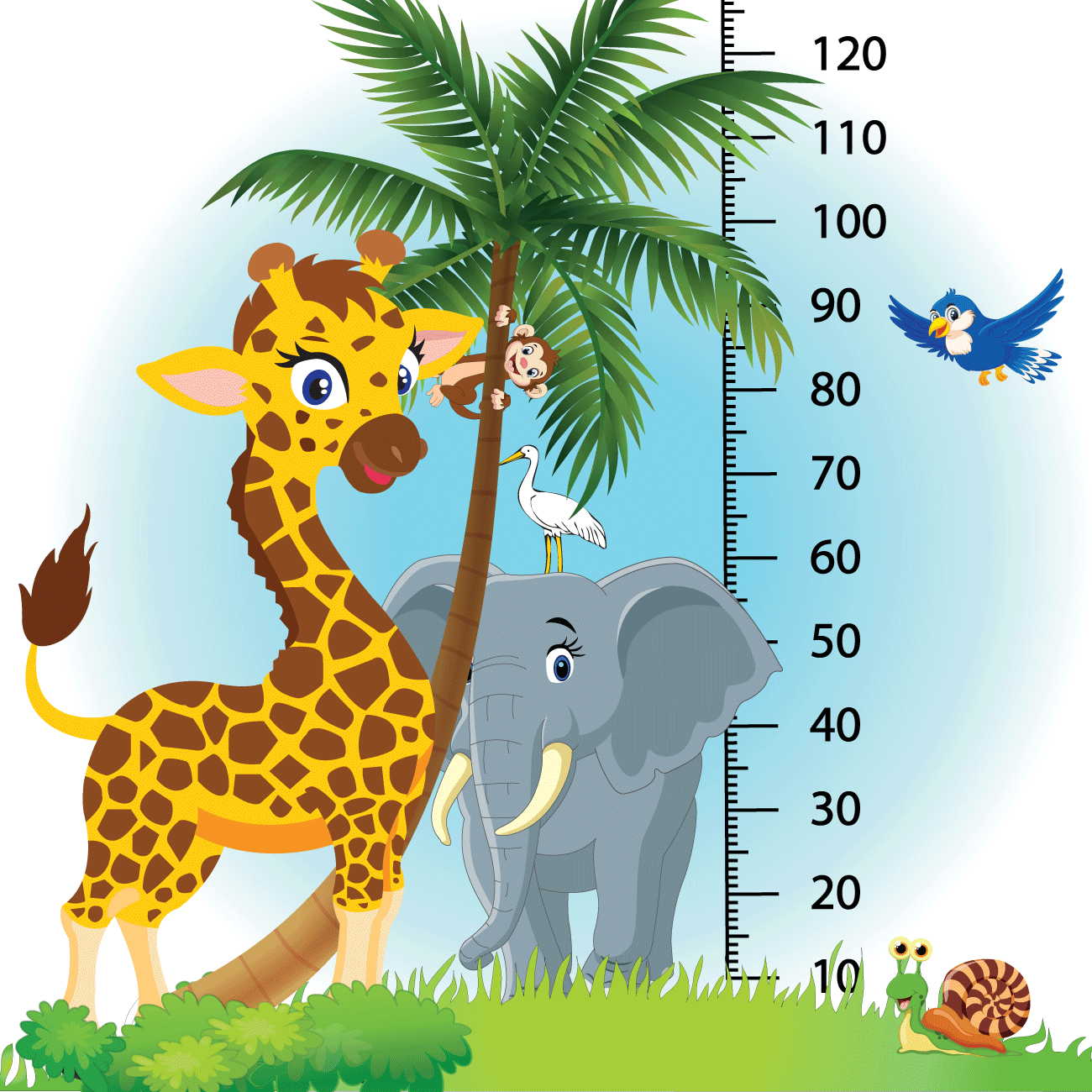Keep your class focused with this batch of printable measurement worksheets that ticks off the list of essential elementary math topics. Understand measurable attributes of objects such as weight, height, capacity, time, and money and solve problems involving measurement and conversion of measurements.

### Statistics and Data Worksheets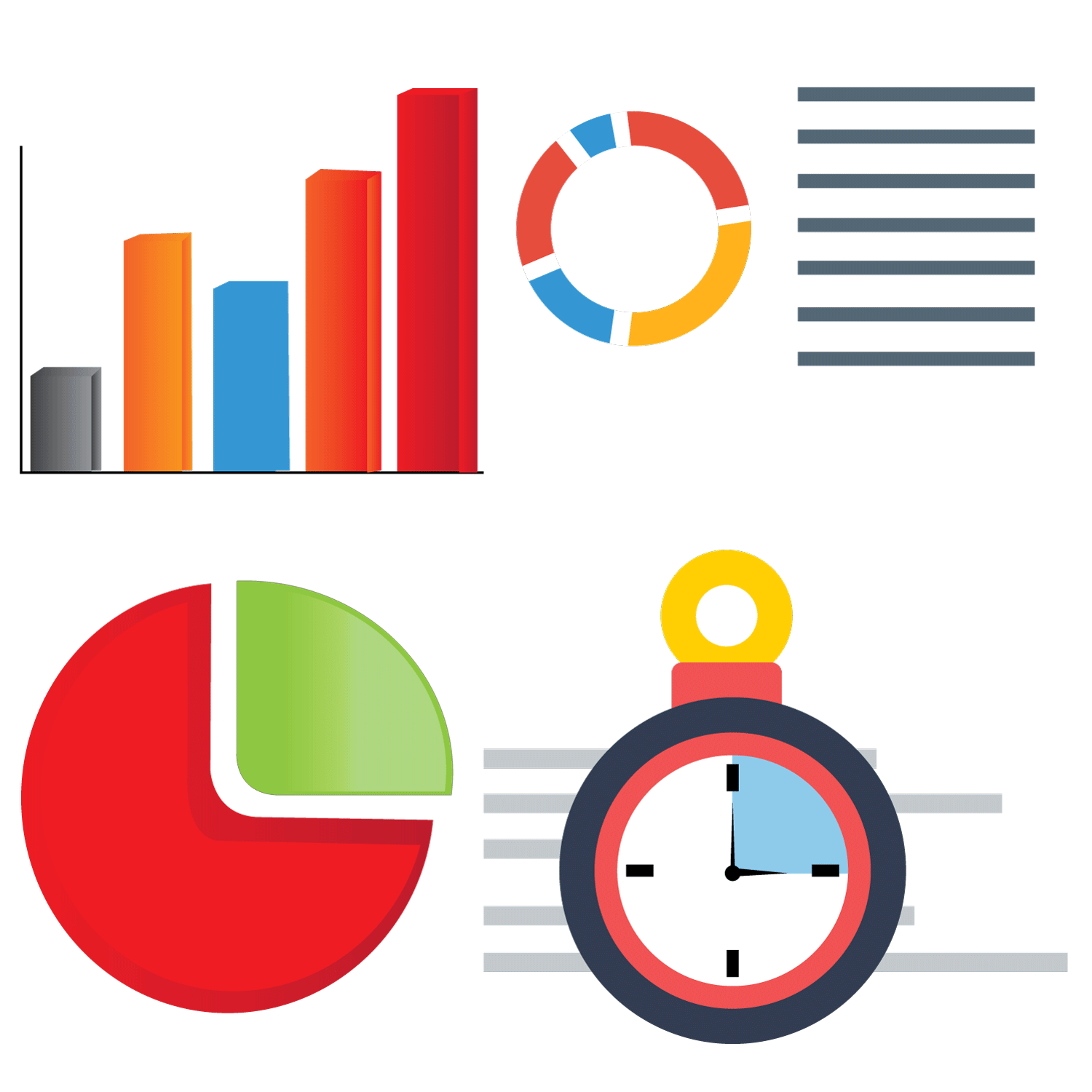Organizing, analyzing, presenting data, and drawing conclusions from them is what this enormous collection of free printable statistics worksheets focuses on. There's a lot more in store for the students to discover in the form of finding the mean, median, mode and range, probability to mention just a few.

### Geometry Worksheets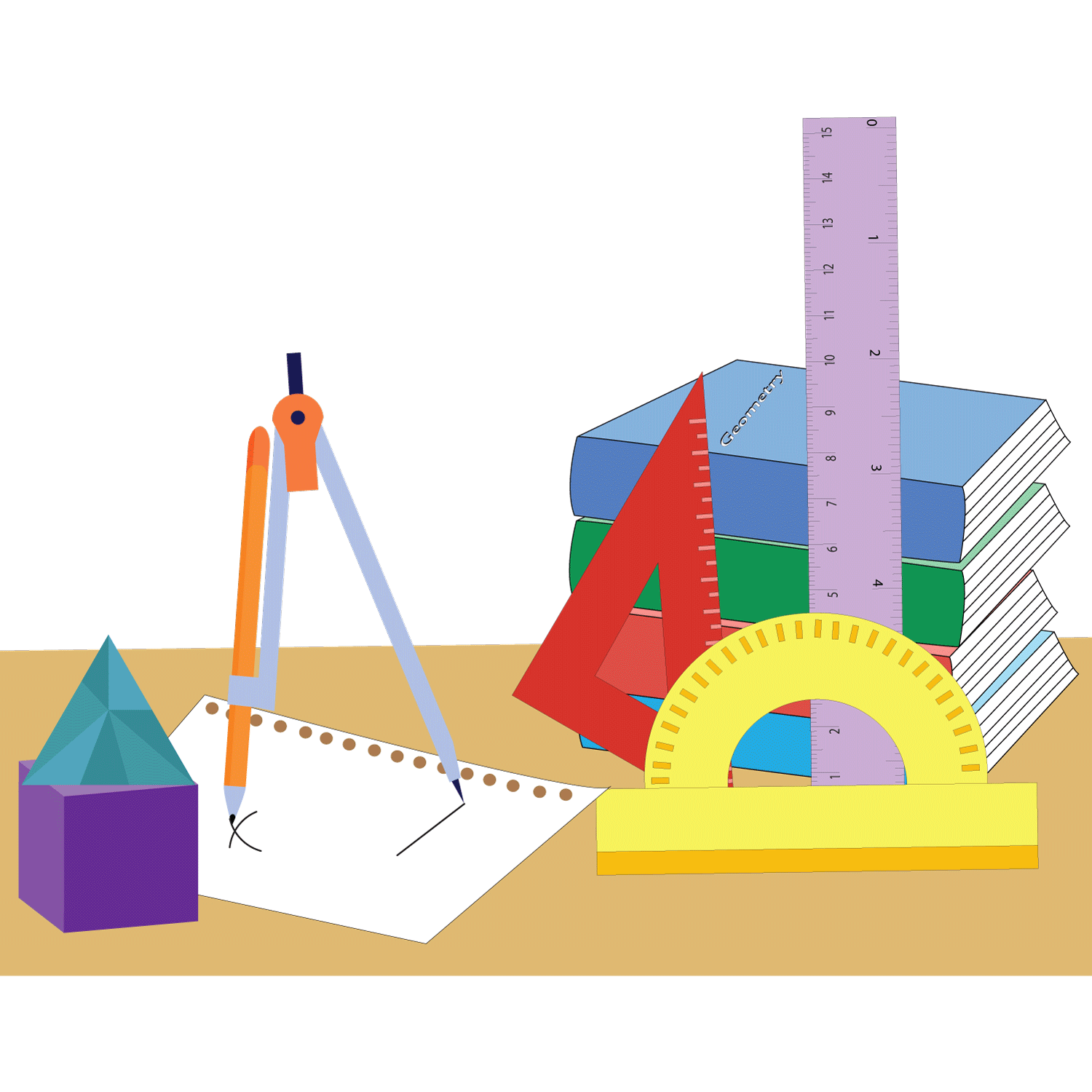Geometry has been a vital part of math, and the fundamental topics are revisited throughout the CCSS math curriculum. Use our free printable geometry worksheets for students in kindergarten through grade 12 and unravel an organized approach to learning about the shapes and spaces around us.

### Pre-Algebra Worksheets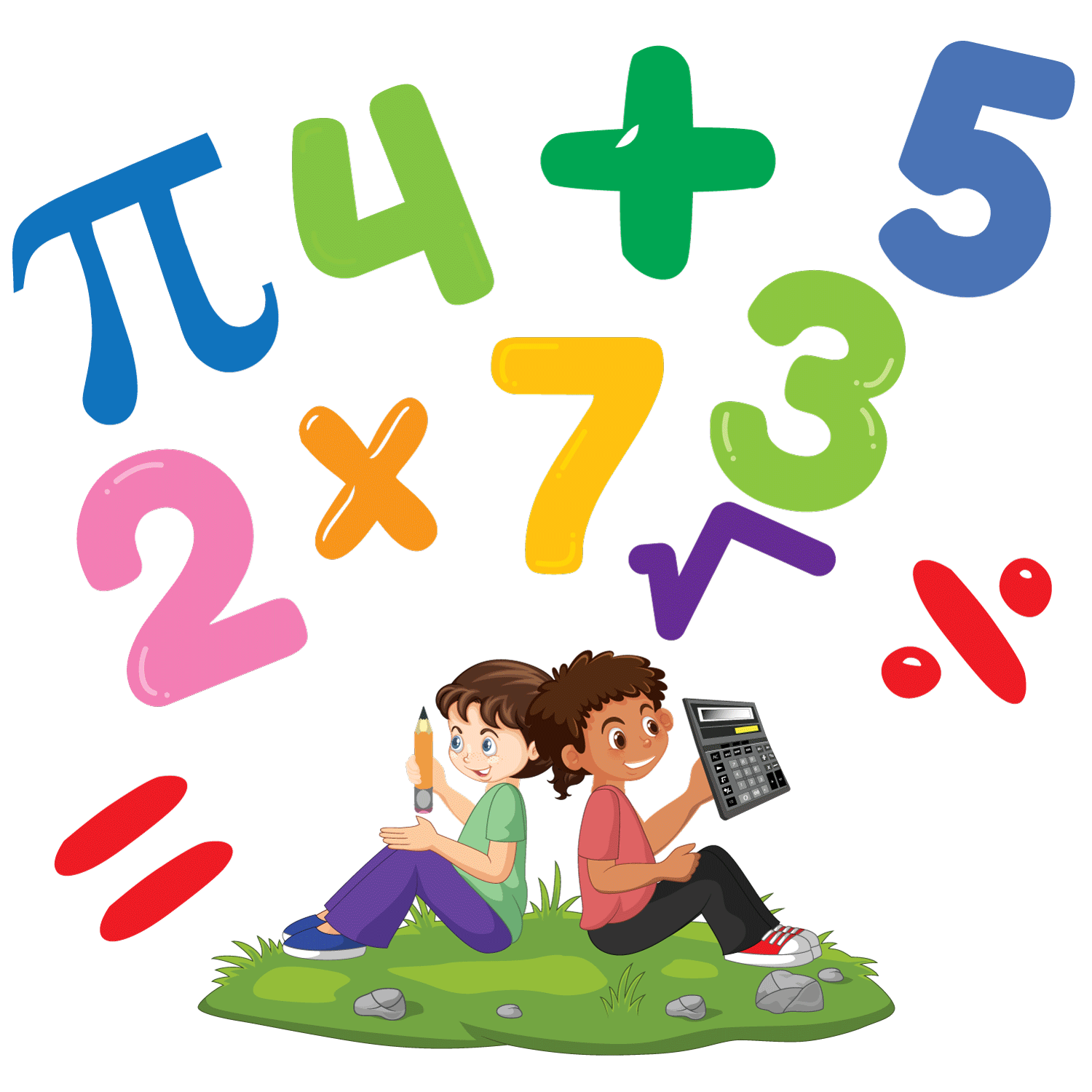The free pre-algebra printable worksheets are oriented toward understanding patterns, factors and multiples; representing and analyzing algebraic expressions, using number types like decimals, integers, fractions, comprehending ratios, proportions, and more.

### Algebra Worksheets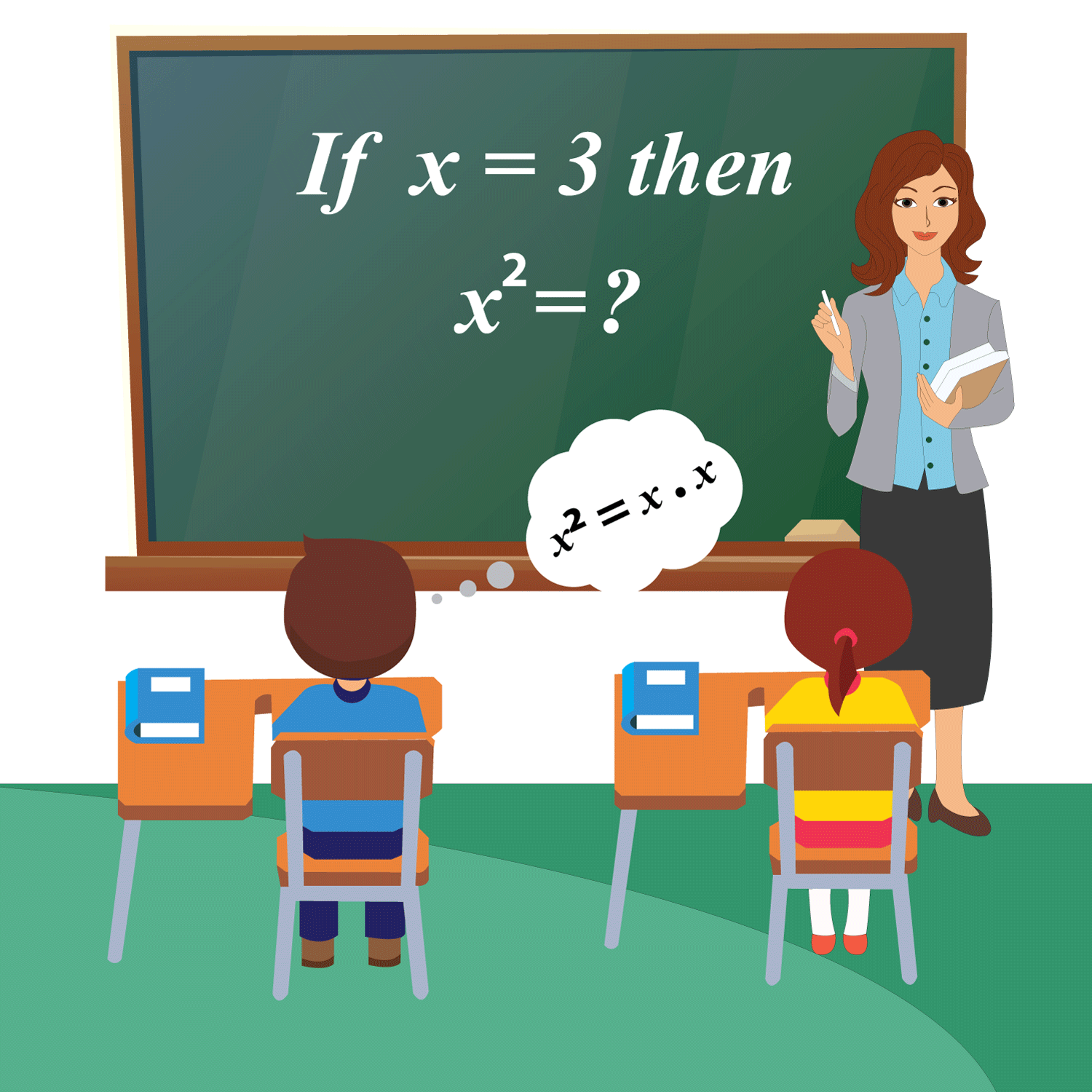Symbols and rules to manipulate the symbols are what we are going to discuss in this section of our free printable algebra worksheets. Excel in interpreting expressions, converting verbal descriptions to equations, writing expressions in equivalent forms to solve problems, and much more.